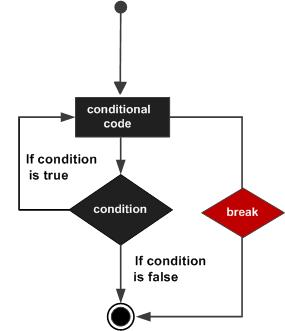# MATLAB - The break Statement

The break statement terminates execution of for or while loop. Statements in the loop that appear after the break statement are not executed.

In nested loops, break exits only from the loop in which it occurs. Control passes to the statement following the end of that loop.

## Flow Diagram## Example

Create a script file and type the following code −

```a = 10;
% while loop execution
while (a < 20 )
fprintf('value of a: %d\n', a);
a = a + 1;
if( a > 15)
% terminate the loop using break statement
break;
end
end
```

When you run the file, it displays the following result −

```value of a: 10
value of a: 11
value of a: 12
value of a: 13
value of a: 14
value of a: 15
```
matlab_loops.htm